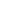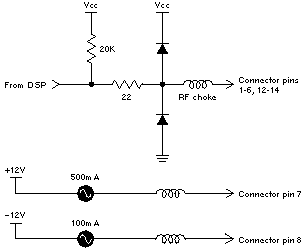E

Details of the DSP

 Memory Map

 The following table describes the memory map for the DSP private RAM (8K words).

 Start End Name p:0 p:\$1FF On-chip program RAM (`\$' denotes hex) p:\$2000 p:\$3FFF Off-chip program RAM, image 1 p:\$A000 p:\$BFFF Off-chip program RAM, image 2 x:0 x:\$FF On-chip data RAM, x bank x:\$100 x:\$1FF On-chip data ROM, x bank (Mu-Law, A-law tables) x:\$2000 x:\$3FFF Off-chip data RAM, x bank, image 1 x:\$A000 x:\$AFFF Off-chip data RAM, x bank, image 2 y:0 y:\$FF On-chip data RAM, y bank y:\$100 y:\$1FF On-chip data ROM, y bank (Sine wave cycle) y:\$2000 y:\$3FFF Off-chip data RAM, y bank, image 1 y:\$A000 y:\$AFFF Off-chip data RAM, y bank, image 2

 Off-chip memory exists in two "images" for each space.  In image 1, all three memory spaces occupy the same physical memory (in other words, the X/Y~, PS~, and DS~ pins of the DSP56001 are not connected when address line A15 is low).  In image 2, x and y are split into separate 4K banks, and p overlays them both with an 8K image (that is, X/Y~ is used as address line A12 and PS~ and DS~ are not connected when A15 is high).  External memory starts at 8K (\$2000) instead of 512 (\$200) because address line A13 in the DSP must be high to enable external DSP RAM.  (Note that there is another enable for this RAM in the System Control Register 2.)

 DSP D-15 Connector Pinouts

 The following describes the output pins of the DSP D-15 connector at the back of the main unit.  The left column is the connector pin number, and the right column is the signal name as it appears in the Motorola DSP56000/DSP56001 Digital Signal Processor User's Manual.

 D-15 DSP1 SCK2 SRD3 STD4 SCLK5 RXD6 TXD7 +12V, 500mA812V, 100mA9 GND10 GND11 GND12 SC213 SC114 SC015 GND

 Figure E-1 shows the circuit through which signals are sent from the DSP to the D-15 connector.Figure E-1.  D-15 Connector There's a series RF choke on each connector signal that doesn't affect its steady-state level.

 DSP56001 Instruction Set Summary

 The following notation is used in the summary:

 Notation Denotes `*' Instructions that don't allow parallel data moves [a,b] One of a or b Either a,b or b,a A nonnegative integer #I n-bit immediate value A n-bit absolute address An A0, A1, or A2  (similarly for Bn) Xn X0 or X1  (similarly for Yn) Rn R0, R1, R2, R3, R4, R5, R6, or R7  (similarly for Nn, Mn) AnyEa Addressing modes (Rn)[[Nn]], (Rn+Nn),(Rn)  (similarly for An) AnyXY [x,y]:AnyEa AnyIO [x,y]:<

 left-justified moves:[A,B,Xn,Yn] right-justified moves:[An,Bn,Rn,Nn] Arithmetic Instructions
 ABS [A,B] Absolute Value ADC [X,Y],[A,B] Add Long with Carry ADD [X,Xn,Y,Yn,B,A],[A,B] Add ADDL [B,A],[A,B] Shift Left then Add  (D=2*D+S) ADDR [B,A],[A,B] Shift Right then Add  (D=D/2+S) ASL [A,B] Arithmetic Shift Left  (D1=D1*2) ASR [A,B] Arithmetic Shift Right  (D1=D1/2) CLR [A,B] Clear Accumulator CMP [Xn,Yn,B,A],[A,B] Compare  (CCR=Sign(D1S)) CMPM [Xn,Yn,B,A],[A,B] Compare magnitude  (CCR=Sign(DS)) *DIV [Xn,Yn],[A,B] Divide Iteration  (D/S iteration) MAC[Xn,Yn],[Xn,Yn],[A,B] Signed Multiply-Add  (no X1*X1, Y1*Y1) MACR[Xn,Yn],[Xn,Yn],[A,B] Signed Multiply, Accumulate, and Round MPY[Xn,Yn],[Xn,Yn],[A,B] Signed Multiply  (no X1*X1, Y1*Y1) MPYR[Xn,Yn],[Xn,Yn],[A,B] Signed Multiply-Round  (no X1*X1, Y1*Y1) NEG [A,B] Negate Accumulator *NORM [A,B] Normalize Accumulator Iteration RND [A,B] Round Accumulator SBC [X,Y],[A,B] Subtract Long with Carry  (D = DSC) SUB [X,Xn,Y,Yn,B,A],[A,B] Subtract  (D = DS) SUBL [B,A],[A,B] Shift Left then Subtract  (D = 2*DS) SUBR [B,A],[A,B] Shift Right then Subtract  (D = D/2S) *Tcc [Xn,Yn,B,A],[A,B] Transfer Conditionally TFR [Xn,Yn,B,A],[A,B] Transfer Data ALU Register TST [A,B] Test Accumulator

 Logical Instructions
 AND [Xn,Yn],[A,B] Logical AND  (D1=D1&S) *ANDI #I8,[MR,CCR,OMR] AND Immediate with Control Register EOR [Xn,Yn],[A,B] Logical Exclusive OR  (D1=D1 XOR S) LSL [A,B] Logical Shift Accumulator Left  (D1=D1<<1) LSR [A,B] Logical Shift Accumulator Right  (D1=D1>>1) NOT [A,B] Logical Complement on Accumulator  (D1=~D1) OR [Xn,Yn],[A,B] Logical Inclusive OR  (D1=D1S) *ORI #I8,[MR,CCR,OMR] OR Immediate with Control Register ROL [A,B] Rotate Accumulator Left  ([C,D1] ROL) ROR [A,B] Rotate Accumulator Right  ([D1,C] ROR)

 Bit Manipulation Instructions
 *BCLR #B5,AnyXY Bit Test and Clear  (C = Selected bit) *BSET #B5,AnyXY Bit Test and Set  (C = Selected bit) *BCHG #B5,AnyXY Bit Test and Change  (C = Selected bit) *BTST #B5,AnyXY Bit Test on Memory  (C = Selected bit) *JCLR #B5,[AnyXY,AnyIO],xxxx Jump if Bit Clear *JSET #B5,[AnyXY,AnyIO],xxxx Jump if Bit Set *JSCLR #B5,[AnyXY,AnyIO],xxxx Jump to Subroutine if Bit Clear *JSSET #B5,[AnyXY,AnyIO],xxxx Jump to Subroutine if Bit Set

 Loop Instructions
 *DO [[x,y]:[AnyEa,A12],AnyReg],L Start Hardware Loop  (L=Label after end) *ENDDO Exit from Hardware Loop

 Move Instructions
 *LUA (Rn)[[Nn]],[Rn,Nn] Load Updated Register MOVE (NOP) Move Data *MOVEC Move Control Register *MOVEC [#I16,#I8],Creg Move Control Register *MOVEC Move Control Register *MOVEM Move Program Memory *MOVEP <[AnyReg,AnyXY],AnyIO> Move Peripheral Data *MOVEP #I24,AnyIO Move Peripheral Data

 Program Control Instructions
 *Jcc [A12,AnyEa] Jump Conditionally *JMP [A12,AnyEa] Jump *JScc [A12,AnyEa] Jump to Subroutine Conditionally *JSR [A12,AnyEa] Jump to Subroutine *NOP No Operation *REP [AnyXY,#I12,AnyReg] Repeat Next Instruction *RESET Reset Peripherals RTI Return from Interrupt RTS Return from Subroutine *STOP Stop Processing *SWI Software Interrupt *WAIT Wait for Interrupt